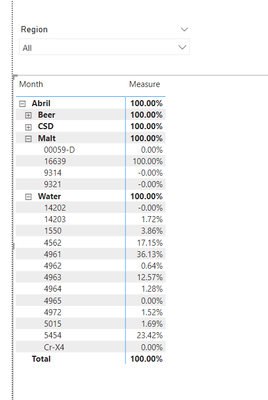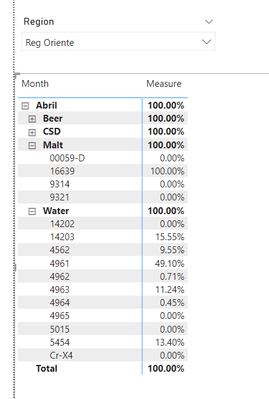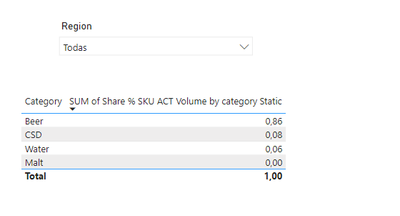cancel
Showing results for
Did you mean:Helper II

## DAX CALCULATE ERROR

Hello, I have the following problem.

I have a measure built in DAX called Share % SKU ACT Volume, it is the percentage participation that each SKU has in the total ACT Volume, with that measure I have no problem.

Now I need to build a SUMIF that calculates the Share % SKU ACT Volume grouped by category for which I am using CALCULATE. So far I managed to build a code that brings me the correct result but when I apply the region filter or any filter, the result is static.

my code is as follows:

``SUM of SKU ACT Volume Share % by Category Static = CALCULATE(Worksheet[SKU ACT Volume % Share], ALLEXCEPT(Worksheet, Worksheet[Month], Worksheet[Category]))``

I understand that the result is static because I am using ALLEXCEPT, so he tried to set my CALCULATE in such a way that my result changes and is correct according to the applied filters.

So I come up with the following code:

``SUM of Share % SKU ACT Volume by category = CALCULATE(Worksheet[Share % SKU ACT Volume], ALLSELECTED(Worksheet), VALUES(Worksheet[Month]), VALUES(Worksheet[Category]))``

The result I get is incorrect, my intention is to get to the result of the measure SUM of SKU ACT Volume Share % by Category Static but that the result changes according to the applied filters.

How can I achieve it?

Share PBI File PBI FILE

1 ACCEPTED SOLUTIONHelper II

I solved it like this:

``````VAR Numerator = CALCULATE(SUM(Worksheet[ACT Volume]), ALLSELECTED(Worksheet), VALUES(Worksheet[Month]),VALUES(Worksheet[Category]))

VAR Denominator = CALCULATE(SUM(Worksheet[ACT Volume]), ALLSELECTED(Worksheet))

RETURN

DIVIDE(Numerator, Denominator)
``````

5 REPLIES 5Helper II

I solved it like this:

``````VAR Numerator = CALCULATE(SUM(Worksheet[ACT Volume]), ALLSELECTED(Worksheet), VALUES(Worksheet[Month]),VALUES(Worksheet[Category]))

VAR Denominator = CALCULATE(SUM(Worksheet[ACT Volume]), ALLSELECTED(Worksheet))

RETURN

DIVIDE(Numerator, Denominator)
``````Solution Specialist

you can't calculate a colnum, it need to be a measure, like sum the colnum or ave the colnum

``SUM of SKU ACT Volume Share % by Category Static = CALCULATE(Sum(Worksheet[SKU ACT Volume % Share]), ALLEXCEPT(Worksheet, Worksheet[Month], Worksheet[Category]))``Helper II

A calculated column would contain static values ​​and I need my result to change as I apply filters on the report.

To add the measure I must create another measure with a SUMX to force the context of the row but before doing so I must obtain the correct values ​​from my calculateSolution Specialist

OKOK

So... you want calculate ACT Volume Share % for each SKU ID base on colnum Month and colnum Category. And sum all those number become 100%

like this``````Measure = AVERAGEX(
VALUES(Worksheet[index]),
CALCULATE(
DIVIDE(
SUM([ACT Volume]),
CALCULATE(
SUM([ACT Volume]),
REMOVEFILTERS(Worksheet[SKU ID])
)
)
)
)``````
``index = [Month]&[Category]``Helper II

Thanks for your answer. It is not what I need, the participation percentage is already calculated, which is the Share % SKU ACT Volume measure, now what I have to do is, that measure, which is my participation percentage, include it in a calculate that groups it by month and category .

So if you can see with the following code:

``SUM of Share % SKU ACT Volume by category Static = CALCULATE(Worksheet[Share % SKU ACT Volume], ALLEXCEPT(Worksheet, Worksheet[Month], Worksheet[Category]))``

Result

Without filtersIt has the result that I expect, what happens is that this result is not dynamic since I apply the region filter and it is the same all the time, what I need is to get that result and that it fits to the granularity defined by the filters.

With filtersThe expected result would be the distribution of 100% that represents the total of my participation among my four categories and that this be dynamic every time I change the region filter.